The sides of a triangle are 5 cm, 12 cm and 13 cm, then its area is

# The sides of a triangle are 5 cm, 12 cm and 13 cm, then its area is

1. A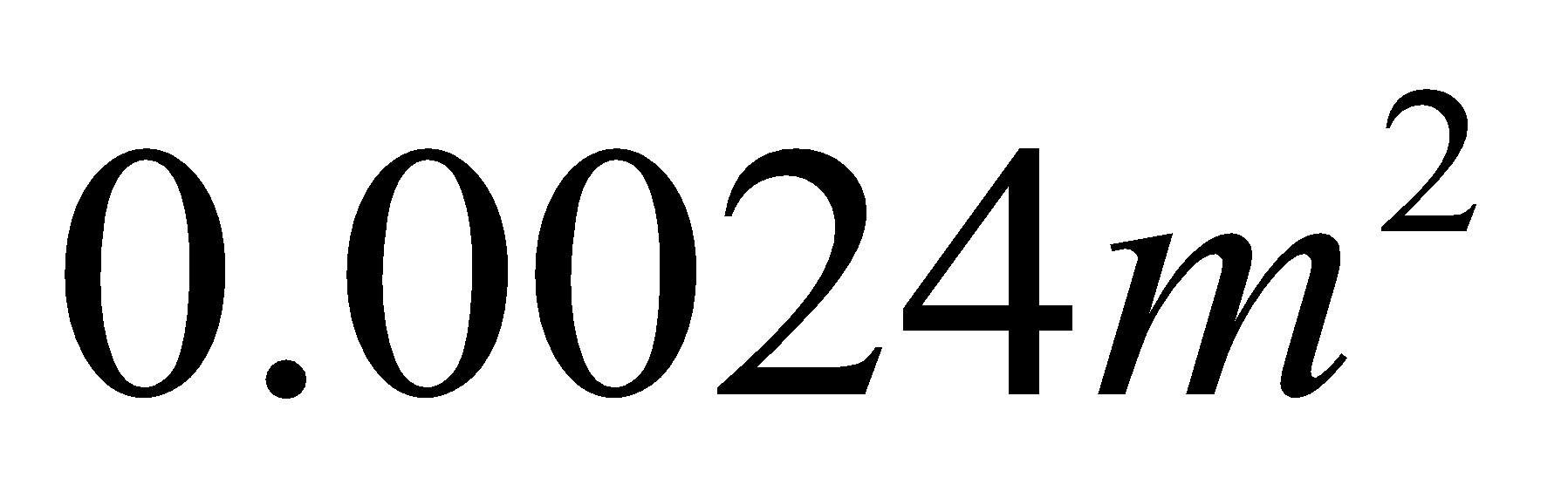2. B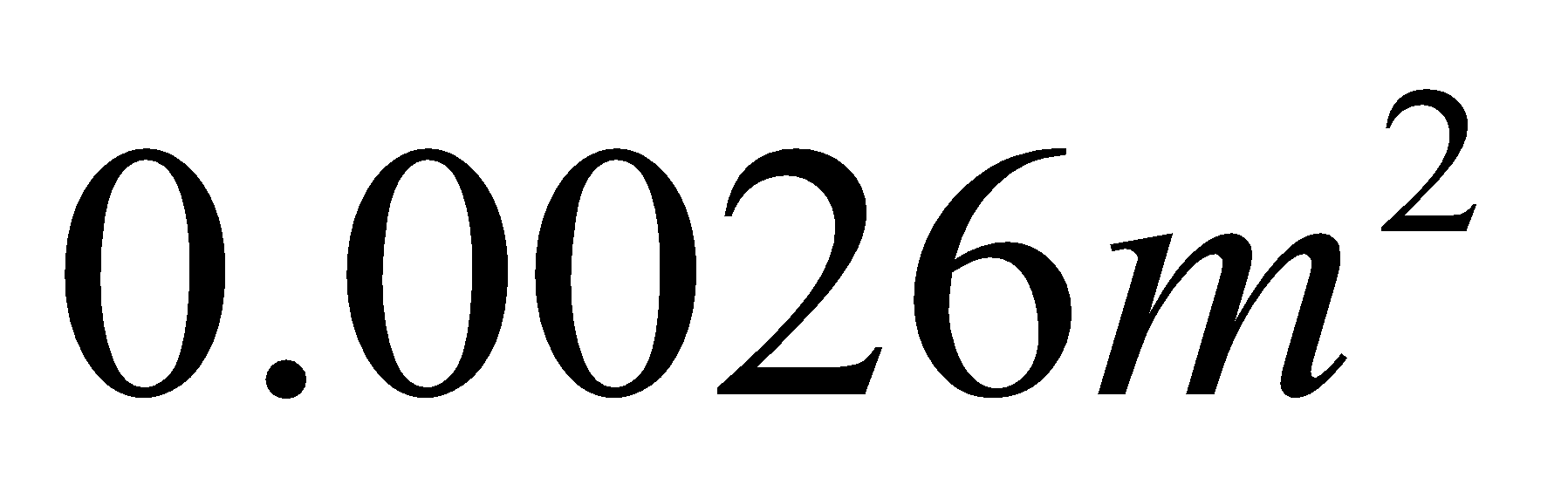3. C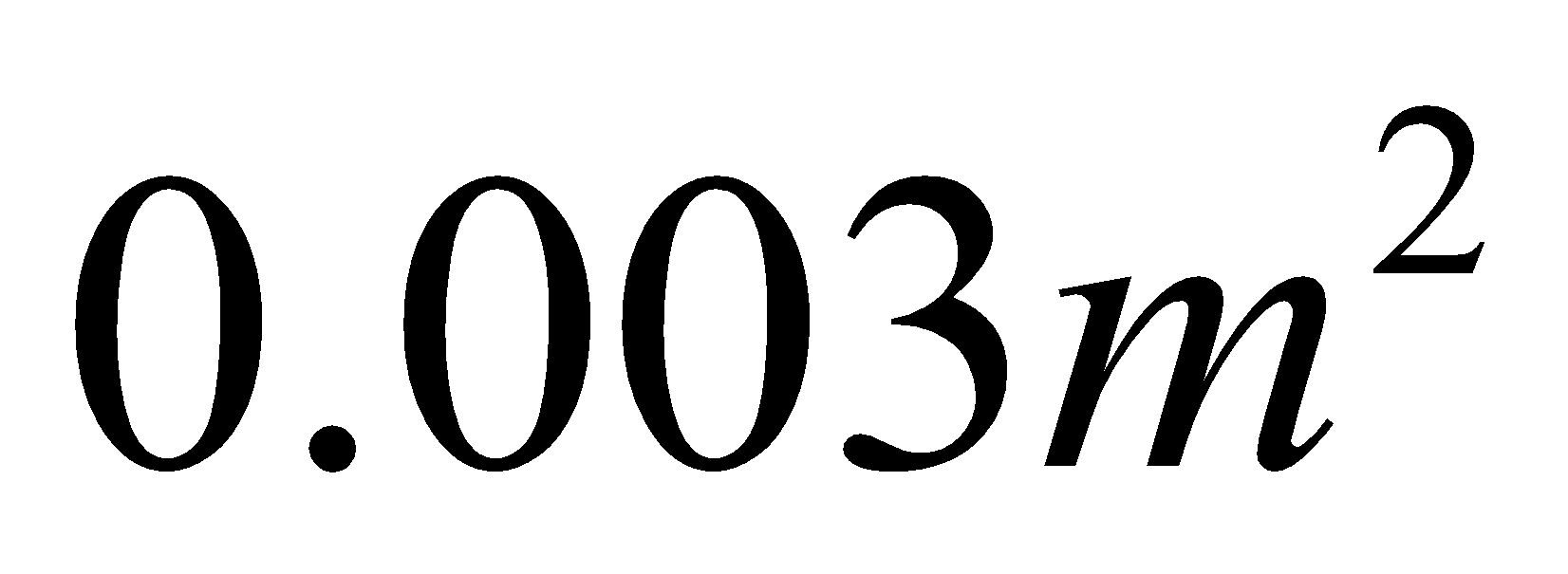4. D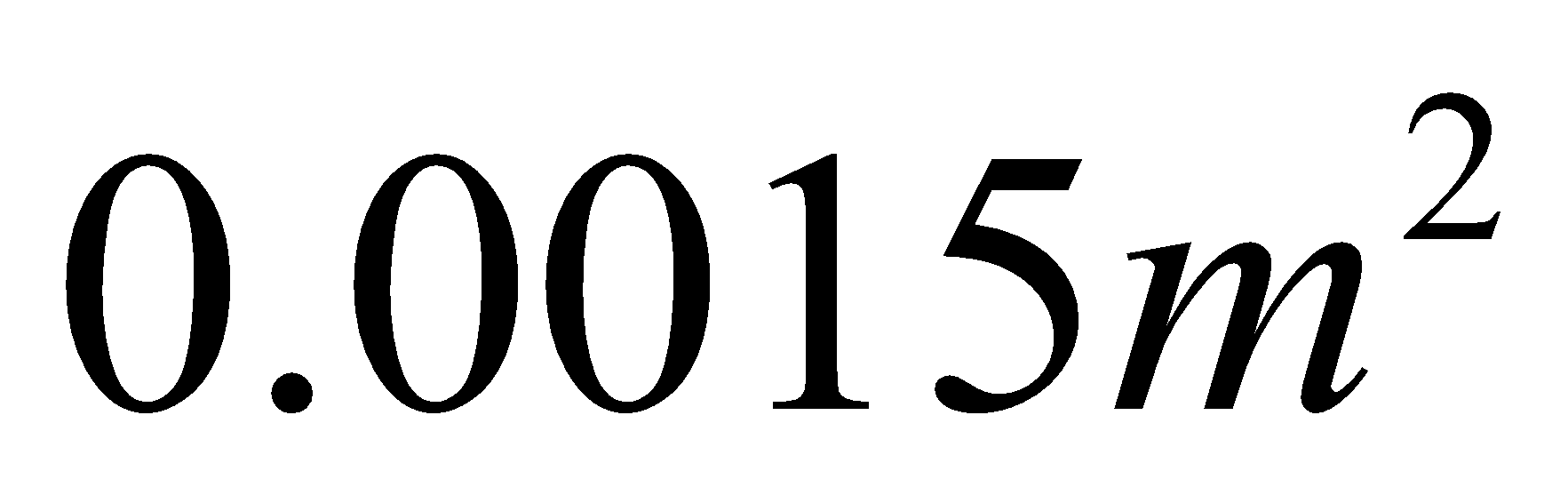Fill Out the Form for Expert Academic Guidance!l

+91

Live ClassesBooksTest SeriesSelf Learning

Verify OTP Code (required)

### Solution:

Here, we using Heron's formula,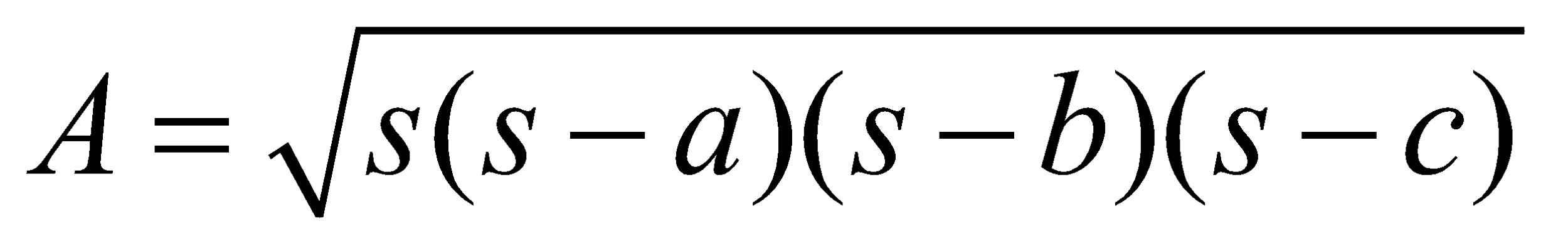Where,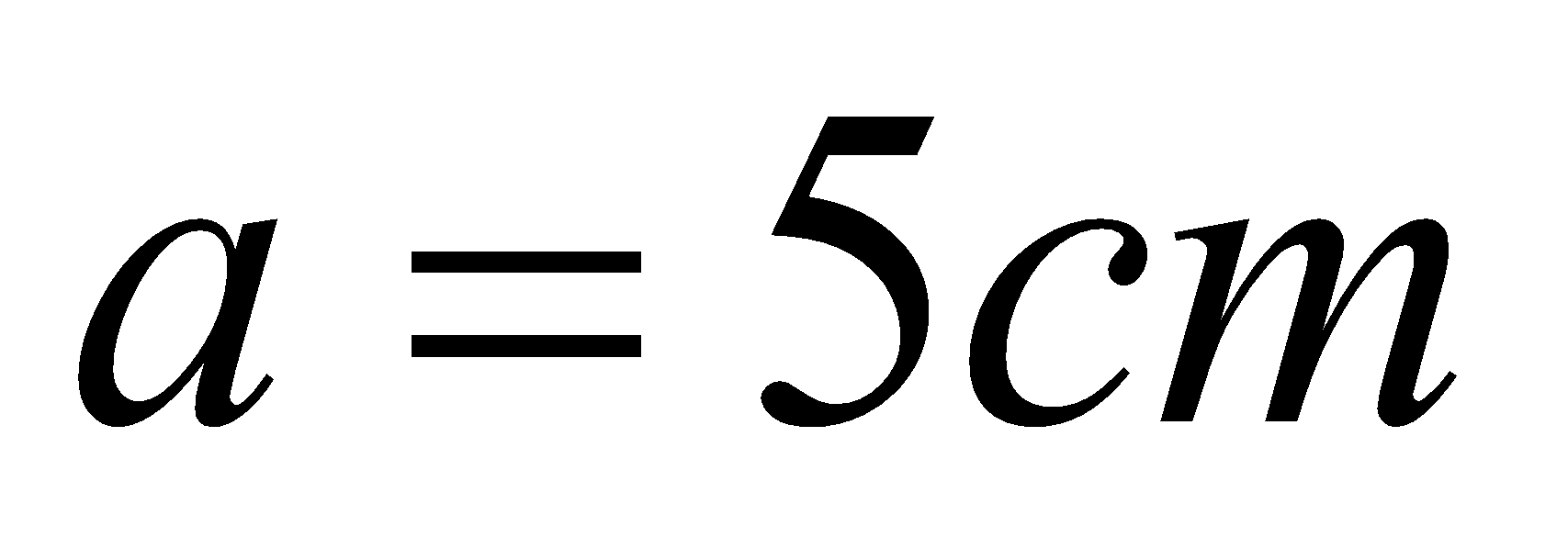,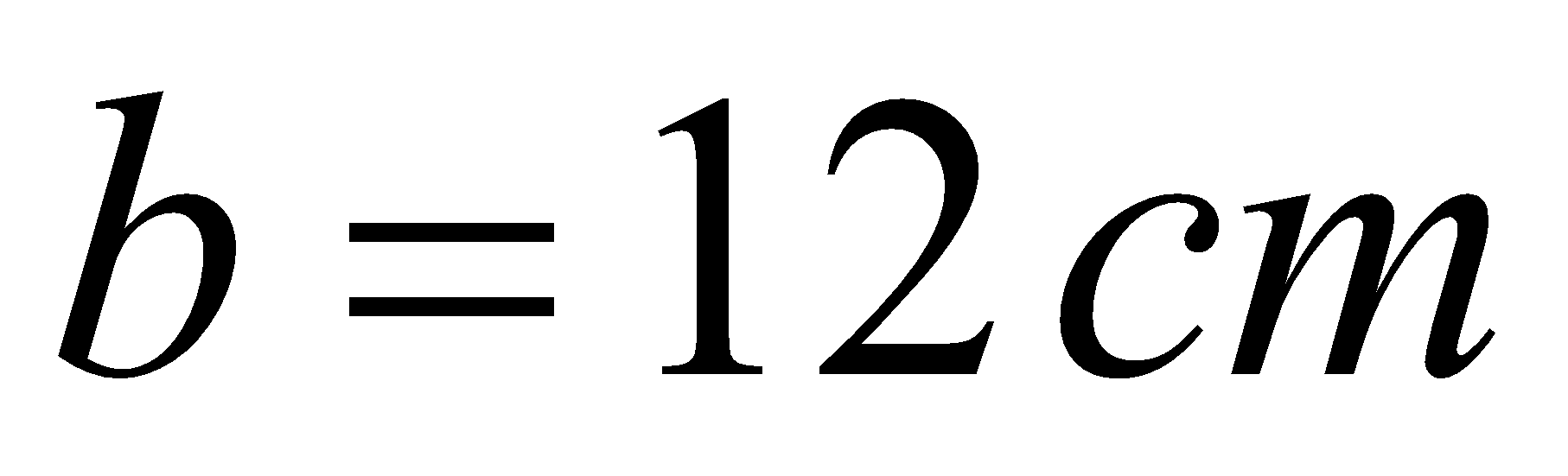and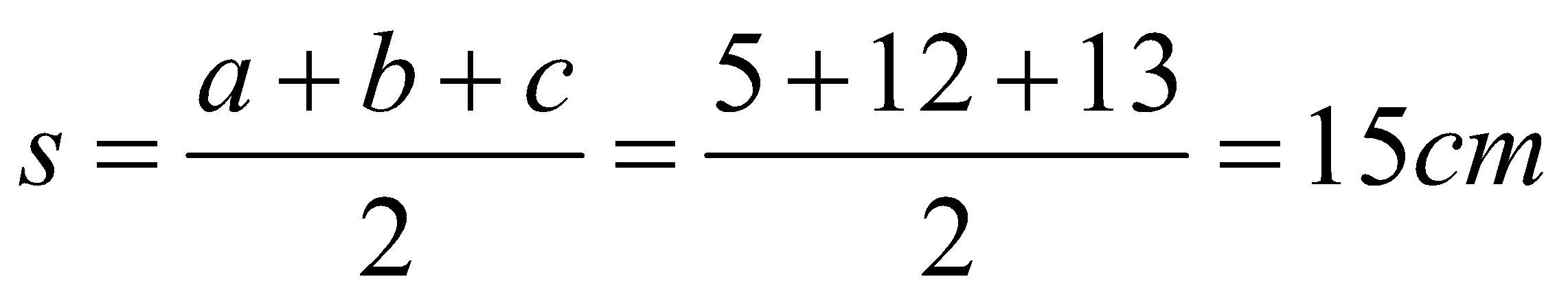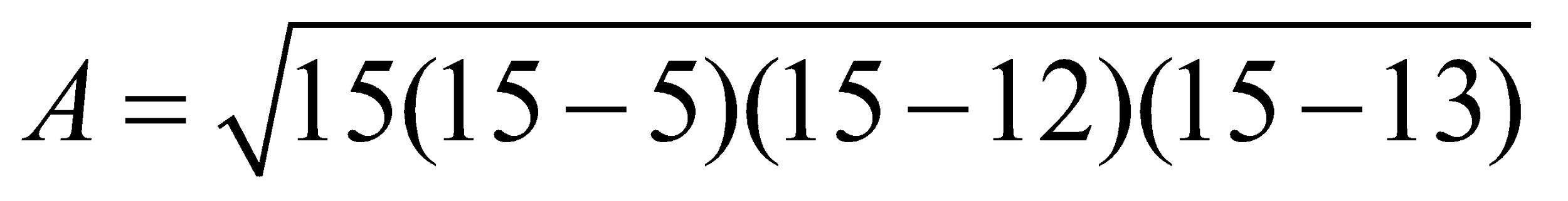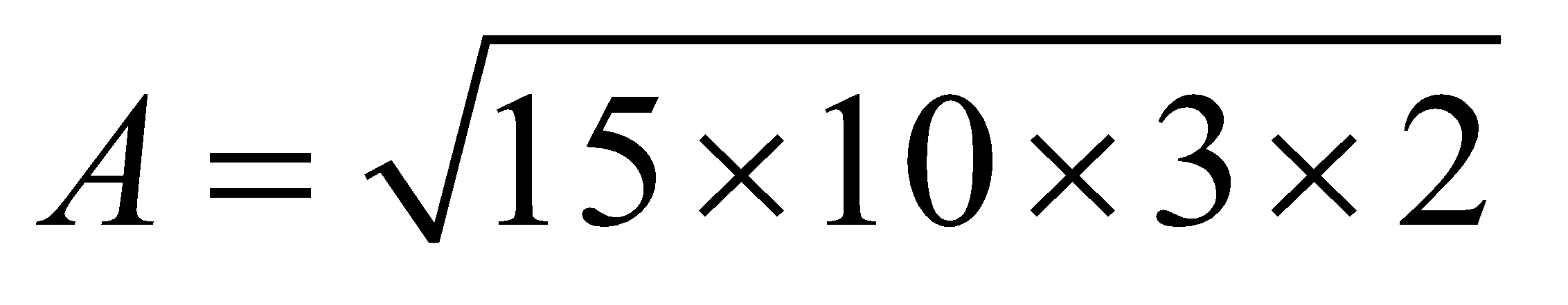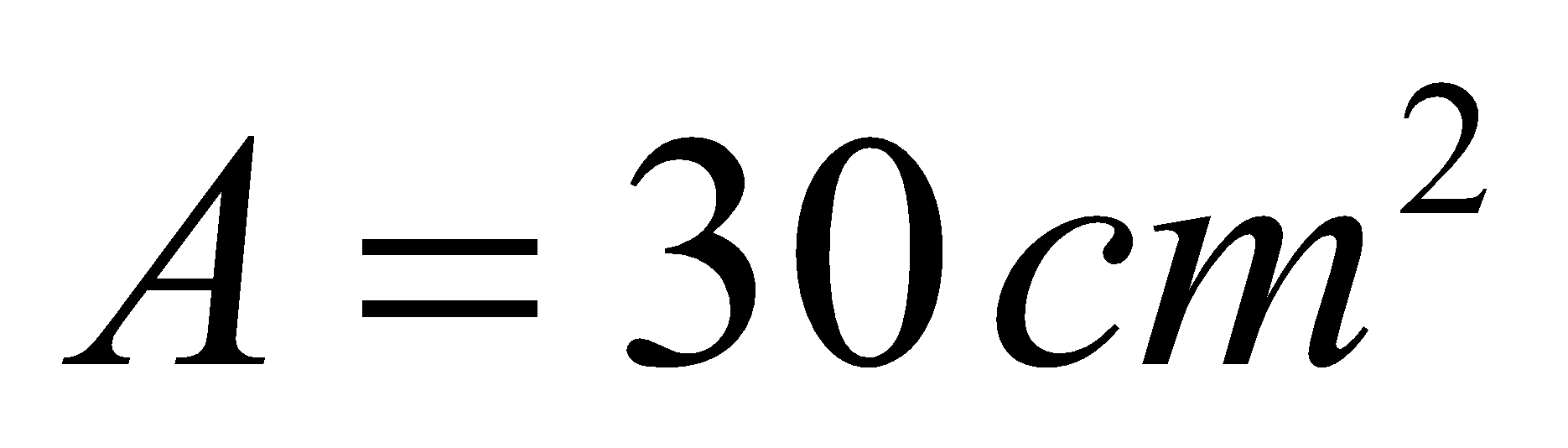Thus,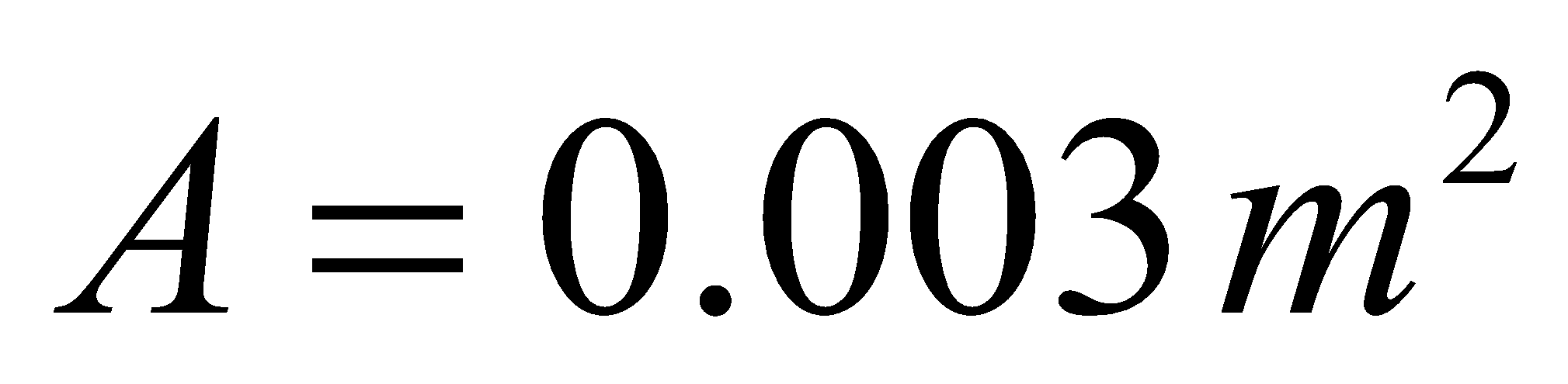Hence, Option (3) is the correct answer.

## Related content

 Area of Square Area of Isosceles Triangle Pythagoras Theorem Triangle Formula Perimeter of Triangle Formula Area Formulae Volume of Cone Formula Matrices and Determinants_mathematics Critical Points Solved Examples Type of relations_mathematics+91

Live ClassesBooksTest SeriesSelf Learning

Verify OTP Code (required)